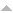# Publications

Found 2290 results
Author Title Type [ Year]
1989
Equations for pseudovarieties. In: Formal properties of finite automata and applications (Ramatuelle, 1988). Vol 386. Springer, Berlin; 1989. 1. p. 148-164p. (Lecture Notes in Comput. Sci.; vol 386).Edit
The join of the pseudovarieties of $\scr R$-trivial and $\scr L$-trivial monoids. J. Pure Appl. Algebra. 1989;60:129-137.Edit
On power varieties of semigroups. J. Algebra. 1989;120:1-17.Edit
Residually finite congruences and quasi-regular subsets in uniform algebras. Portugal. Math.. 1989;46:313-328.Edit
Semidirect products of pseudovarieties from the universal algebraist's point of view. J. Pure Appl. Algebra. 1989;60:113-128.Edit
1990
A constructive method for constructing integral convolutions. Dokl. Akad. Nauk BSSR. 1990;34:588-591, 666.
An elementary proof that finite groups are projectively torsion-free. Portugal. Math.. 1990;47:437-444.Edit
The equation $\bf PX=\bf PJ$. In: Proceedings of the International Symposium on the Semigroup Theory and its Related Fields (Kyoto, 1990). Shimane Univ., Matsue; 1990. 1. p. 1-11p. Edit
Local controllability in $3$-manifolds. Systems Control Lett.. 1990;14:45-49.
On pseudovarieties, varieties of languages, filters of congruences, pseudoidentities and related topics. Algebra Universalis. 1990;27:333-350.Edit
Semidirectly closed pseudovarieties of locally trivial semigroups. Semigroup Forum. 1990;40:315-323.Edit
Simplicial convex $4$-polytopes do not have the isotopy property. Portugal. Math.. 1990;47:309-318.Edit
Some two-dimensional integral transformations of convolution type. Dokl. Akad. Nauk BSSR. 1990;34:396-398, 474.Edit
1991
Algebraic varieties characterizing matroids and oriented matroids. Adv. Math.. 1991;87:160-185.Edit
The Arrhenius plot of a physiological rate process is never linear. Ciência e Cultura. 1991;43(5):363-369.Edit
On direct product decompositions of finite $\scr J$-trivial semigroups. Internat. J. Algebra Comput.. 1991;1:329-337.Edit
On finite simple semigroups. Proc. Edinburgh Math. Soc. (2). 1991;34:205-215.Edit
The generalizations of integral analog of the Leibniz rule on the $G$-convolutions. Extracta Math.. 1991;6:119-122.Edit
Generalizations of the Leibniz rule to integral convolutions. Dokl. Akad. Nauk BSSR. 1991;35:111-115, 188.Edit
Generating operators and convolutions for some integral transformation. Dokl. Akad. Nauk BSSR. 1991;35:773-776, 860.Edit
Geometric conditions for local controllability. J. Differential Equations. 1991;89:388-395.
Implicit operations on finite $\scr J$-trivial semigroups and a conjecture of I. Simon. J. Pure Appl. Algebra. 1991;69:205-218.Edit
Integral convolutions for $H$-transformations. Izv. Vyssh. Uchebn. Zaved. Mat.. 1991:72-79.Edit
Integral convolutions of Laplace type for $G$-transforms. Vests\=ı Akad. Navuk BSSR Ser. F\=ız.-Mat. Navuk. 1991:11-16, 123.
On iterated semidirect products of finite semilattices. J. Algebra. 1991;142:239-254.Edit

Error | CMUP

# Error

The website encountered an unexpected error. Please try again later.